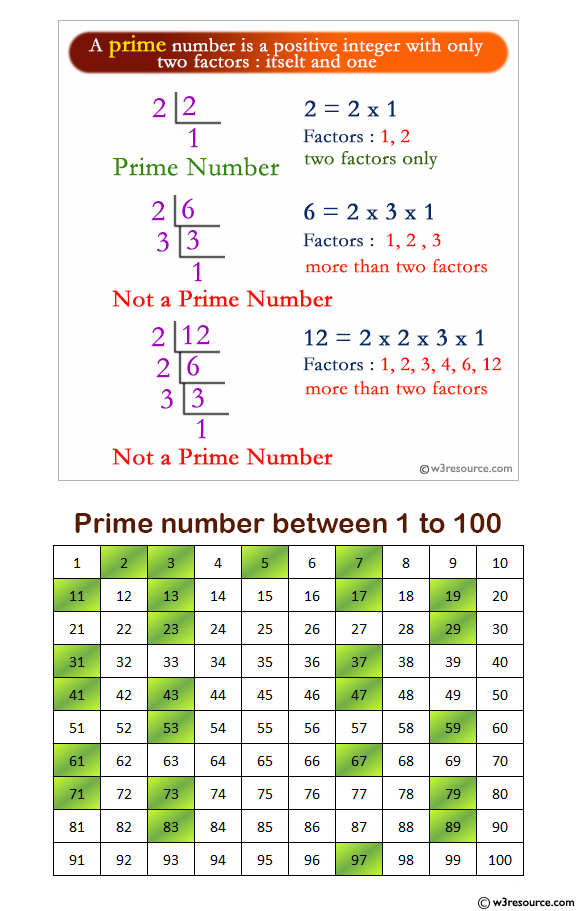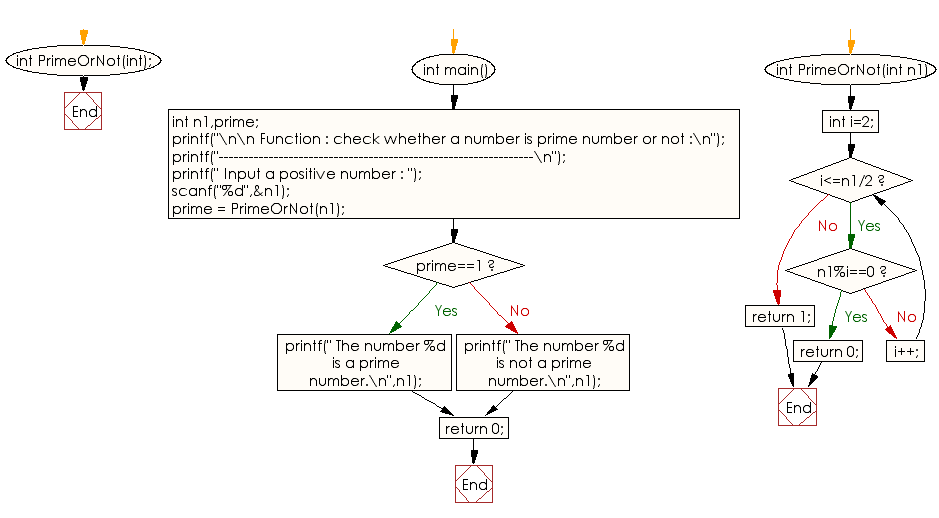﻿ C Program: Check whether a number is a prime number or not - w3resource

# C Exercises: Check whether a number is a prime number or not

## C Function : Exercise-7 with Solution

Write a program in C to check whether a number is a prime number or not using the function.

Pictorial Presentation:Sample Solution:

C Code:

``````#include<stdio.h>
int PrimeOrNot(int);
int main()
{
int n1,prime;
printf("\n\n Function : check whether a number is prime number or not :\n");
printf("---------------------------------------------------------------\n");

printf(" Input a positive number : ");
scanf("%d",&n1);
prime = PrimeOrNot(n1);
if(prime==1)
printf(" The number %d is a prime number.\n",n1);
else
printf(" The number %d is not a prime number.\n",n1);
return 0;
}
int PrimeOrNot(int n1)
{
int i=2;
while(i<=n1/2)
{
if(n1%i==0)
return 0;
else
i++;
}
return 1;
}
```
```

Sample Output:

``` Function : check whether a number is prime number or not :
---------------------------------------------------------------
Input a positive number : 5
The number 5 is a prime number.
```

Explanation:

```int PrimeOrNot(int n1) {
int i = 2;
while (i <= n1 / 2) {
if (n1 % i == 0)
return 0;
else
i++;
}
return 1;
}
```

The function 'PrimeOrNot' takes a single argument 'n1' of type int. It checks whether the input number 'n1' is prime or not by iterating through all integers from 2 to n1/2. The function initializes a local integer variable i to 2 and enters a while loop that continues as long as i is less than or equal to n1/2. In each iteration of the loop, the function checks if 'n1' is divisible by i. If it is, the function returns 0, indicating that n1 is not a prime number. Otherwise, i is incremented by 1 and the loop continues. If the loop completes without finding any divisors of 'n1', the function returns 1, indicating that n1 is a prime number.

Time complexity and space complexity:

The time complexity of this function is O(n), where n is the input number n1, because the while loop iterates n1/2 - 2 + 1 times.

The space complexity of this function is O(1), as it only uses a fixed amount of memory to store the single integer variable i.

Flowchart:C Programming Code Editor:

What is the difficulty level of this exercise?

Test your Programming skills with w3resource's quiz.

﻿